# RBSE Maths Class 10 Chapter 10: Locus Important Questions and Solutions

RBSE Class 10 Maths Chapter 10 – Locus Important questions and solutions are available here. All the questions provided are aimed at a better understanding of students. The RBSE Class 10 important questions and solutions given at BYJU’S has a full coverage of all the topics from the textbooks and will help the students in mastering these topics.

Chapter 10 of RBSE Class 10 has only two exercises which have questions of finding the locus of a set of points in different situations. Several theorems are available here along with the detailed proofs. Enough questions are provided here which cover all the concepts, which are important for the exams. This chapter helps the students in higher classes while dealing with more geometry related topics.

### RBSE Maths Chapter 10: Exercise 10.1 Textbook Important Questions and Solutions

Question 1: State whether the following statements are true or false and justify your answer.

(i) The set of the points equidistant from the given line is also a line.

(ii) A circle is a locus of the point which is equidistant from a certain point.

(iii) Three points are collinear only when they are the elements of the set of the points of a line.

(iv) The locus of the points equidistant from two lines will be a parallel line.

(v) The locus of a point equidistant from two given points, the perpendicular bisector of the line joining the two points.

Solution:

(i) False, the locus of the points equidistant from the given line are parallels on either side of this line.

(ii) True, the fixed point is the centre and fixed distance is the radius.

(iii) False, three points may collinear only when they are the elements of the set of points which lie on that line.

(iv) False, it depends on the position of two lines (if two given lines are parallel, then it will be parallel to them and if they intersect each other then it will be the bisector of angle formed by them).

(v) True, the locus of a point equidistant from two given points, the perpendicular bisector of the line joining the two points.

Question 2: The diagonals of a quadrilateral bisect each other. Prove that the given quadrilateral is a parallelogram.

Solution:

ABCD is a quadrilateral ABCD whose diagonals AC and BD bisect each other at O.

Thus, OA = OC and OB = OD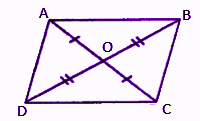In ΔAOB and ΔCOD,

OA = OC (given)

∠AOB = ∠COD (vertically opposite angles)

OB = OD (given)

BY ASA congruence rule,

ΔAOB ≅ ΔCOD

⇒ ∠OAB = ∠OCD (by CPCT)

⇒ ∠CAB = ∠ACD

These are alternate angles formed by a transversal of lines AB and CD.

That means AB || CD.

Therefore, ABCD is a parallelogram.

Hence proved.

Question 3: What will be the locus of a point equidistant from three non-collinear points A, B, and C? Justify your answer.

Solution:

The locus of points will be the center of the circle.

Justification:

Let us take three non-collinear points A, B and C (on a circle).

Join AB and BC.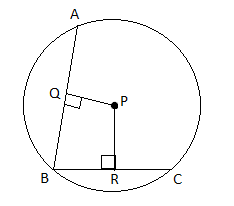Let P be a moving point which is equidistant from A and B.

PQ is the perpendicular bisector of AB.

Similarly, P is equidistant from B and C. Also, PR is the perpendicular bisector of BC.

Thus, P is the intersecting point of perpendicular bisectors of AB and BC.

Therefore, P is the center of the circle which passes through the three given non-collinear points.

Question 4: What will be the locus of a points equidistant from three collinear points? Justify your answer.

Solution:

Let A, B, and C be the three collinear points on the line l.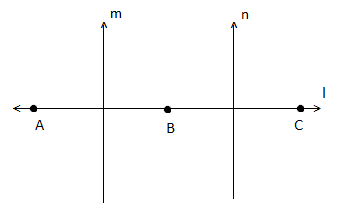m and n be the perpendicular bisectors.

Any point which is equidistant from points A and B lies on the perpendicular bisector of AB, i.e on the line m.

Similarly, any point which is equidistant from points B and C lies on line n which is the perpendicular bisector of BC.

Similarly, any point which is equidistant from points A, B and C lie on a common perpendicular of two lines m and n.

Here, m ⊥ AB and n ⊥ BC

⇒ m ⊥ AC and n ⊥ AC

⇒ m || n

Thus, there is no common point in m and n.

Therefore, there is no point which is equidistant from three collinear points.

Hence, these points did not exist.

Question 5: Prove that the locus of the centres of the circles passing through the points A and B is the bisector of the line segment AB.

Solution:

Let A and B be the centers of two circles C1 and C2, each of these passing through two given points P and Q.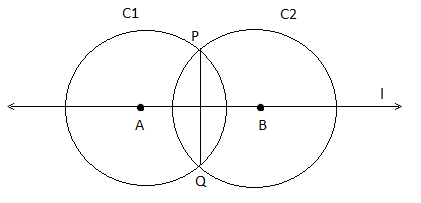Then, PA = QA (radii of the circle C1)

⇒ A lies on the perpendicular bisector of PQ ….(i)

Again PB = QB (radii of the circle C2)

⇒ B lies on the perpendicular bisector of PQ ….(ii)

From (i) and (ii),

A, B lie on the perpendicular bisector of PQ.

Hence, the locus of the centers of all the circles passing through P and Q is the perpendicular bisector of PQ.

Question 6: In the figure, ∆PBC and ∆QBC are situated on the opposite sides of common base BC. Prove that the line joining P and Q is the perpendicular bisector of BC.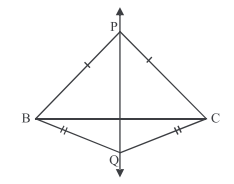Solution:

Given,

∆PBC and ∆QBC are two isosceles triangles which lie on both sides of base BC.

Thus, PB = PC and BQ = CQ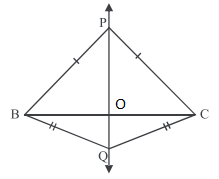In ∆PBC,

PB = PC (given)

∴ ∠PBO = ∠PCO (angles opposite to equal sides)

PO = PO (common)

By SAS congruence rule,

∆POB ≅ ∆POC

By CPCT,

∠PBO = ∠POC ….(i)

We know that,

∠PBO + ∠POC = 180°

∠PBO + ∠POB = 180° [From (i)]

2∠POB = 180°

∠POB = 180°/2

∠POB = 90°

∠PBO = ∠POC = 90°

Similarly,

∠QOB = ∠QOC = 90°

Therefore, PQ, bisects BC at 90°

Hence proved.

Question 7: In the figure, ΔPQR and ΔSQR are on the same side of common base QR. Prove that line SP is the perpendicular bisector of QR.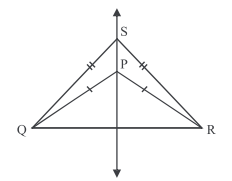Solution:

Given,

Two isosceles triangles PQR and SQR have the same base QR.

Also, SQ = SR and PQ = PR

Since, ∆PQR is an isosceles triangle.

∠PQ = PR

∠PQR = ∠PRQ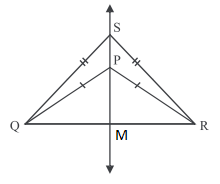In the isosceles triangle, angles opposite to equal sides are also equal.

In ∆PQM and ∆PRM,

PQ = PR (given)

∠PQR = ∠PRQ

PM = PM (common)

By SAS congruence rule,

∆PQM ≅ ∆PRM

By CPCT,

∴ ∠QMP = ∠RMP

QM = MR

Now,

∠QMP + ∠RMP = 180° (linear pair)

∠QMP = ∠RMP = 90°

Thus, PM ⊥ QR

∴ M is the midpoint of QR.

Similarly, the perpendicular bisector of QR is PM.

Point P lies on SM.

∵ ∆SQR is an isosceles triangle.

Also, the perpendicular bisector of QR is SM.

Therefore, line segment SP will be a perpendicular bisector of base QR.

Question 8: In the given figure, PS is the bisector of the angle ∠P intersect QR at S. SN ⊥ PQ and SM ⊥ PR are drawn. Prove that SN = SM.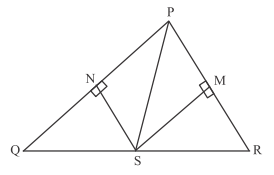Solution:

Given,

In ∆PQS, PS is the bisector of ∠P.

SN ⊥ PQ and SM ⊥ PR.

In ∆PSN and ∆PSM

PS = PS (common)

∠PNS = ∠PMS (each equal to 90° because SN ⊥ PQ and SM ⊥ PR)

∠NPS = ∠MPS (PS is the bisector of ∠P)

By AAS congruence rule

∆ASP ≅ ∆PSM

By CPCT,

SN = SM

Hence proved.

Question 9: In the figure, find the locus of the points inside the ∠ABC and equidistant from two sides BA and BC.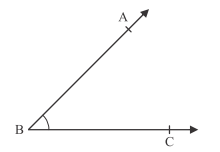Solution:

Construct an angular bisector BX of ∠ABC.

Take any point P on BX and draw perpendiculars on AB and BC such that PL ⊥ AB and PM ⊥ BC.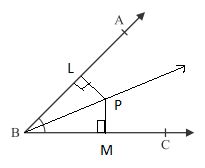∴ ∠PLB = ∠PMB = 90°

In ∆PLB and ∆PMB,

∠PLB = ∠PMB (by construction)

∠LBP = ∠PBM (BP is bisector of ∠B)

BP = BP (common)

BY AAS congruence rule,

∴ ∆PLB ≅ ∆PMB

By CPCT,

PL = PM

Therefore, point P is the interior of ∠ABC and equidistant from AB and BC is required locus.

### RBSE Maths Chapter 10: Exercise 10.2 Textbook Important Questions and Solutions

Question 10: Find the locus of a point equidistant from the three vertices and also find the locus of a point equidistant from the sides of a triangle.

Solution:

Let ABC be the triangle and O be the moving point which is equidistant from three vertices A, B, and C.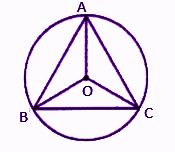O is equidistant from A and B.

That means O is the perpendicular bisector of AB.

O is equidistant from A and C.

That means O is the perpendicular bisector of AC.

Thus, O is the intersecting point of perpendicular bisectors of AB and AC. Therefore, O is the center of the circle, passes through three vertices and O is called the circumcenter of the circle.

Hence, the required locus will be the circumcenter of the circle.

Let O be the point which is equidistant from path D, E, and F of sides BC, AC and AB, respectively.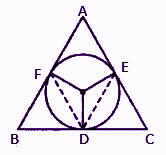Here, O is equidistant from D and E.

That means O is the bisector of DE.

O is equidistant from point R.

That means O is the perpendicular bisector of DF.

Thus, O is the intersecting point of DE and DF.

Therefore, O is the centre of a circle touching three sides of triangle and O is the incentre.

Hence, the required locus is the in-centre of the circle.

Question 11: In the triangle ABC, medians AD, BE and CF intersect each other at G. If AG = 6 cm, BE = 9 cm, and GF = 4.5 cm, then find the length of GD, BG and CF.

Solution:

Given,

In triangle ABC, medians AD, BE and CF intersect each other at G.

AG = 6 cm, BE = 9 cm, and GF = 4.5 cm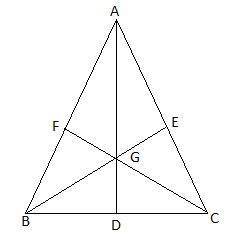We know that the centroid of the triangle divides its medians in the ratio 2 : 1.

AG/GD = 2/1

6/GD = 2/1

⇒ GD = 6/2

⇒ GD = 3 cm

BG/GE = 2/1

BG/(BG + GE) = 2/(2 + 1)

BG/BE = 2/3

BG/9 = 2/3

BG = (2/3) × 9

BG = 6 cm

CG/GF = 2/1

(CG + GF)/GF = (2 + 1)/1

CF/GF = 3

CF/4.5 = 3

CF = 4.5 × 3

CF = 13.5 cm

Therefore, GD = 3 cm, BG = 6 cm and CF = 13.5 cm

Question 12: In a triangle, medians AD, BE and CF intersect each other at G, prove that AD + BE > (3/2)AB.

[Hint: AG + BG > AB]

Solution:

Let ABC be the triangle in which medians AD, BE and CF intersect each other at G.Given that, AG + BG > AB

Thus, the sum of two sides of a triangle is greater than the third side.

We know that the centroid of the triangle divides its medians in the ratio 2 : 1.

AG/GD = 2/1

AG/(AG + GD) = 2/(2 + 1)

Similarly,

BG = (2/3)BE

AG + BG > AB (given)

Hence proved.

Question 13: In a triangle ABC, prove that the sum of two medians is greater than the third one.

Solution:

Let ABC be the triangle in which medians AD, BE and CF intersect each other at G.

Produce AD upto K so that AG = GK.

Join KB and KC.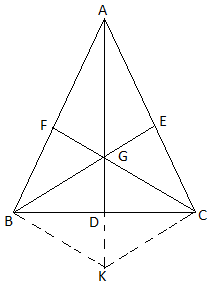In triangle ABK,

F is the midpoint of AB. (given)

G is the midpoint of AK. (by construction)

We know that the line joining the midpoint of two lines of triangle is parallel to the third side.

∴ FG || BK

⇒ GC || BK ….(i)

Similarly,

E is the midpoint of AC. (given)

G is the mid-point of AK. (by construction)

∴ GE || KC

⇒ BG || KC ….(ii)

GC || BK [From (i)]

and BG || KC [From (ii)]

Thus, quadrilateral BKCG is a parallelogram.

⇒ BK = CG ….(iii)

We know that the sum of any two sides of a triangle is greater than the third side.

In triangle BKG,

BG + GK > BK

⇒ BG + AG > CG (by construction AG = GK)

BK = CG [From (iii)]

⇒ (2/3) BE + (2/3)AD > (2/3)CF [since AG = (2/3)AD, BG = (2/3)BE and CG = (2/3)CF]

⇒ BE + AD > CF

Similarly,

Hence proved.

Question 14: In a triangle ABC, medians AD, BE and CF passes through the point G. Prove that 4(AD + BE + CF) > 3(AB + BC + CA)

Solution:

Let ABC be the triangle in which medians AD, BE and CF intersect each other at G.We know that the sum of any two sides of a triangle is greater than the third side.

In triangle BGC,

BG + GC > BC

We know that, BG = (2/3) BE and GC = (2/3)CF]

⇒ (2/3) BE + (2/3)CF > BC

⇒ BE + CF > (3/2)BC ….(i)

In triangle AGB,

AG + GB >AB

We know that, AG = (2/3) AD and BG = (2/3)BE]

⇒ (2/3) AD + (2/3)BE > AB

⇒ AD + BE > (3/2)AB ….(ii),

In triangle AGC,

AG + GC > AC

We know that, AG = (2/3) AD and GC = (2/3)CF]

⇒ (2/3) AD + (2/3)CF > AC ….(i)

⇒ AD + CF > (3/2)AC ….(iii),

BE + CF + AD + BE + AD + CF > (3/2)BC + (3/2)AB + (3/2)AC

⇒ 2(AD + BE + CF) > (3/2)(BC + AB + AC)

⇒ 4(AD + BE + CF) > 3(AB + BC + AC)

Hence proved.

Question 15: In triangle ABC, P is the orthocentre. Prove that orthocentre of ΔPBC is A.

Solution:

Given,

P is the orthocentre of ΔABC.

Let AP, BP and CP are extended such that they meet BC, AC and AB at D, E, and F respectively.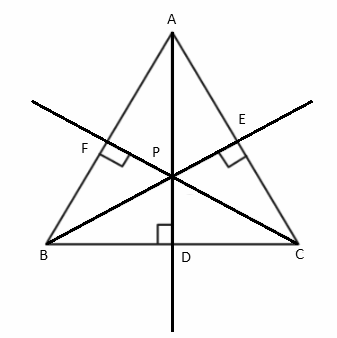Thus, AD ⊥ BC, BE ⊥ AC, CF ⊥ AB

⇒ AD ⊥ BC, AB ⊥ CP, AC ⊥ BP

Therefore, A is the orthocentre of ∆PBC.

Hence proved.

Question 16: In ΔABC, the medians AD, BE and CF pass through the point G.

(a) If GF = 4 cm, then find GC.

(b) If AD = 7.5 cm, then find GD.

Solution:

In ΔABC, the medians AD, BE and CF pass through the point G.We know that the centroid of the triangle divides its medians in the ratio 2 : 1.

(a) Given,

GF = 4 cm

CG/FG = 2/1

CG/4 = 2

CG = 2 × 4

CG = 8 cm

(b) Given,

GD/7.5 = 1/3

GD = 7.5/3

GD = 2.5 cm

Question 17: In an isosceles triangle ABC, AB = AC, and D is the midpoint of BC. Prove that circumcentre, in-centre, orthocentre and centroid all are collinear.

Solution:

In an isosceles triangle ABC, AB = AC, and D is the midpoint of BC.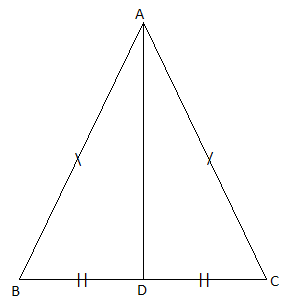In an isosceles triangle, the circumcentre lies on the perpendicular bisector, so we have to show that AD is a perpendicular bisector of the side BC.

∆ABD ≅ ∆ACD (by SSS)

Now,

AD ⊥ BC and BD = CD

⇒ AD is the perpendicular bisector of BC.

Therefore, the circumcentre lies on AD.

For an in-centre of a triangle, we have to prove that AD is bisector of ∠BAC.

∆ABD ≅ ∆ACD [by SSS congruence rule]

⇒ AD is bisector of ∠BAC

For the orthocentre of a triangle, we have to prove that AD is altitude corresponding to side BC.

∆ABD ≅ ∆ACD [by SSS congruence rule]

AD is the perpendicular to BC that means AD is altitude corresponding to side BC.

Hence, the orthocentre lies on AD.

For a centroid of a triangle, we have to prove that AD is median corresponding to side BC.

It is given that D is the midpoint of BC.

AD is the median of triangle ABC.

Hence, the centroid lies on AD.

Hence proved that circumcentre, in-centre, orthocentre and centroid all are collinear.

Question 18: In a triangle ABC, H is the orthocentre. X, Y and Z are midpoints of AH, BH and CH respectively. Prove that orthocentre of ΔXYZ is H.

Solution:

Given,

In a triangle ABC, H is the orthocentre. X, Y and Z are midpoints of AH, BH and CH respectively.

Draw perpendiculars AM, BN and CO are from A, B and C respectively which intersect at H.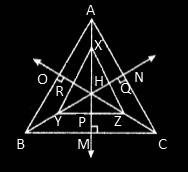In ∆HBC,

BC || YZ

From vertex X, XP is an altitude on YZ.

In ∆AHC,

AC || XZ

From vertex Y, YQ is an altitude on ZX.

Similarly,

In ∆ABH,

AB || XY

From vertex Z, ZR is an altitude on XY.

The above three altitudes intersecting at H.

Therefore, the orthocentre of triangle XYZ will be H.

Hence proved.

Question 19: How will you find a point on side BC of ΔABC which is equidistant from AB and AC?

Solution:

Let us draw a ∆ABC, in which AL, the bisector of ∠A which meets BC at D.

Also, by taking P on AX, draw perpendiculars PN and PM on AB and AC respectively as shown below.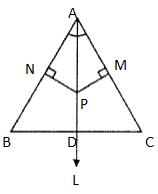PN ⊥ AB and PM ⊥ AC

In ∆APN and ∆APM,

∠PNA = ∠PMA = 90° (by construction)

∠PAN = ∠PAM (AX is the bisector of ∠A)

AP = AP (common)

By AAS congruence rule,

∆APN ≅ ∆APM

⇒ PN = PM (by CPCT)

Hence, P is equidistant from AB and AC.

Therefore, any point on AX will be equidistant from AB and AC.

Thus, D lies on side BC and equidistant from AB and AC.

Hence proved.

### RBSE Maths Chapter 10: Additional Important Questions and Solutions

Question 1: The point equidistant from the vertices of triangle is called

(a) centre of gravitation

(b) circumcentre

(c) orthocentre

(d) incentre

Solution:

In triangle ABC, O is the circumcentre which is equidistant from all the three vertices.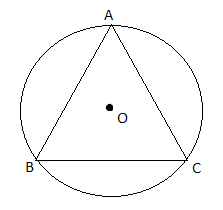Question 2: Gravitation centre of a triangle

(a) Point of concurrency of perpendicular bisectors of the sides of the triangle

(b) concurrent point of angle bisectors of the triangle

(c) point of concurrency of medians of the triangle

(d) the orthocentre

Solution:

Gravitation centre or orthocentre is the point of intersection of medians of the triangle.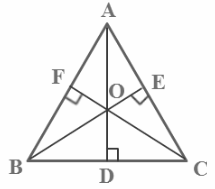Here, O is the orthocentre of triangle ABC.

Question 3: Locus of the center of rolling circle in a plane is:

(A) Circle

(B) Curve

(C) a line parallel to the plane

(D) Perpendicular to the plane

Solution:

The locus of the centre of the rolling circle forms a set of planes from a given point with a two dimensional plane.

Hence, the parallel plane provided by the center of the rolling circle in a plane.

Question 4: If two medians of a triangle are equal, then the triangle is:

(a) right angle

(b) isosceles triangle

(c) equilateral triangle

(d) none of these

Solution:

In an isosceles triangle ABC, AD, BE and CF are medians.

BE = CF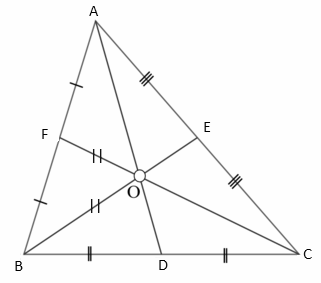Question 5: If AB and CD are two non-parallel lines, then the locus of the point P equidistant from these lines will be:

(a) The line parallel to AB, and passing through the point P

(b) The bisector of the angle subtended by lines AB and CD passing through point P

(c) A parallel line to both AB and CD and passing through point P

(d) The altitude drawn on the sides AB and CD and passing through point P

Solution:

If AB and CD are two non-parallel lines, then the locus of the point P equidistant from these lines will be the bisector of the angle subtended by lines AB and CD passing through point P.

Question 6: The triangle whose orthocentre, circumcentre and incentre coincides is known as

(a) equilateral triangle

(b) right triangle

(c) isosceles triangle

(d) none of these

Solution:

In an equilateral triangle, orthocentre, circumcentre and incentre will coincide as shown below.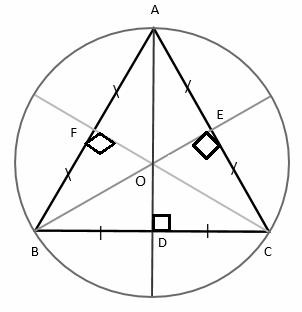Question 7: The triangle whose orthocentre is its vertex point is called

(a) right triangle

(b) equilateral triangle

(c) isosceles triangle

(d) none of these

Solution:

In the right triangle ABC, B is the orthocentre.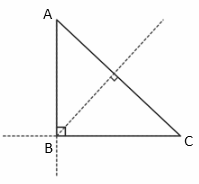Hence, the triangle whose orthocentre is its vertex point is called a right triangle.

Question 8: Find the locus of an end of a pendulum of the clock.

Solution:

When the Pendulum of the clock will be moving, by inspection we can say that the locus of the end of the pendulum will be an arc as shown below.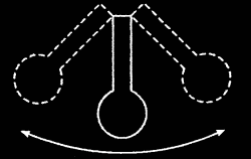Question 9: In a triangle ABC, D, E, and F are the midpoints of side BC, AC and AB respectively, then prove that EF bisects AD.

Solution:

Given,

In a triangle ABC, D, E, and F are the midpoints of side BC, AC and AB respectively.

Join DE, DF and EF.

In triangle ABC,

D and E are midpoints of sides BC and AC respectively.

Therefore, DE || AB

DE = AB

Consider,

DE || AB

⇒ FA || DE ….(i)

Similarly,

EA || DF ….(ii)

From (i) and (ii),

ΔEAF will is a parallelogram.

We know that diagonals of a parallelogram bisects each other.

Hence proved.

Question 10: Find the locus of the points equidistant from the two given points.

Solution:

Let A and B be the two given points.

And P be the point which is equidistant from A and B such that AP = BP.

If D is the midpoint of a line segment joining A and B, then AD = DB.

Thus, D is situated at the locus along with P.

Join PD so that AP and BP become the sides of two triangles ADP and BDP respectively.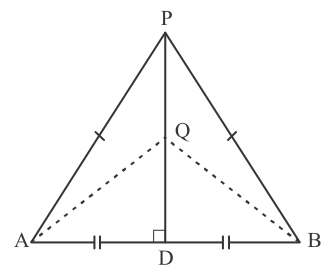By SSS congruence rule,